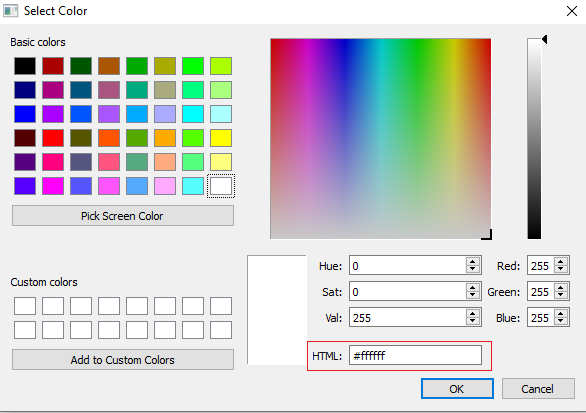# get the RGB hexadecimal color ( QColor)

• Hello guys,
how to get the Hexadecimal RGB color value (see the screenshot) ? thanks• @Zunneh
QColor::rgba()
if you want the string respentation as in your screenshot:

``````QString("#%1").arg(QColor(...).rgba(), 8, 16)
``````

• @raven-worx thank you , only one little problem, i get the Hexadecimal value + ff
for exemple i select #90eeaa but when i click ok i get #ff90eeaa (where this ff come ? )
another question : i want to get the binary value of this hexadecimal , can you help me ? thanks

• @Zunneh
thats the alpha channel. Limit it to 6 characters then.

``````QString("#%1").arg(QColor(...).rgb(), 6, 16)
``````

or compose it by concatenating each color component

``````/QColor c(...);
QString("#%1%2%3").arg(c.red(),2,16).arg(c.green(),2,16).arg(c.blue(),2,16)
``````

• @raven-worx i changed it to 6 but always same problem
i tried also to concetnating each color and convert from decimal to binary but imagine if one color is 0 , then he will write it 0 not 00000000 and the final result will be false

• i changed it to 6 but always same problem

yes i think the second parameter of QString::arg() doesnt limit the output and is only interpreted as minimum length.

@VRonin
does QColor::name() really always return the hex format? I thought i might also return "red" for example.
I cant try myself right now

• @raven-worx it returned the Hex format but in QString, so now i have to convert it to Hex and then to binary (because i want my output in binary )
@VRonin is there a function that returned the color in Hexadecimal and not in a QString ? thanks

• does QColor::name() really always return the hex format? I thought i might also return "red" for example.

`qDebug() << QColor(Qt::red).name();` returns `"#ff0000"`

is there a function that returned the color in Hexadecimal and not in a QString ?

😓 for the numeric representation you can just use `unsigned int colNum = QColor(Qt::red).rgb()`

• it returned the Hex format but in QString, so now i have to convert it to Hex and then to binary (because i want my output in binary )

what is binary in your case?!
QColor::rgb() returns a 32bit value... which contains each color component byte by byte. So its is binary if you want so.

something like this?

``````QByteArray a = QByteArray("#") + QByteArray::number(c.red(),16) + QByteArray::number(c.green(),16) + QByteArray::number(c.blue(),16);
a.toHex()
``````

• i tried this and it work , thanks guys

``````bool ok;
QColor color;
color = QColorDialog::getColor(Qt::white,this);
QString ColorHex=color.name().remove('#');
int ColorInt = ColorHex.toInt(&ok,16);
QString ColorBin= ColorHex.setNum(ColorInt, 2);
lab->setText(ColorBin);``````

• i tried this``````int ColorInt =QColorDialog::getColor(Qt::white,this).rgb()
lab->setText(QString::number(ColorInt ,2));
``````